# 1404 Texas Tessellation

I recently visited family members in Texas. My daughter-in-law is awesome at both mathematics and quilting. My photo does not do her work justice, but Texas is tessellated in this quilt! She also carefully chose the fabrics she pieced together. Do they remind you of anything for which Texas is famous?Someone else designed the pattern, but piecing these pieces together was not the easiest sewing project.

I wondered if anyone else had thought to tessellate Texas and found a couple of examples on twitter. As this first one asked, should we call this Texellation?

Now I’ll tell you something about the number 1404:

• 1404 is a composite number.
• Prime factorization: 1404 = 2 × 2 × 3 × 3 × 3 × 13, which can be written 1404 = 2² × 3³ × 13.
• 1404 has at least one exponent greater than 1 in its prime factorization so √1404 can be simplified. Taking the factor pair from the factor pair table below with the largest square number factor, we get √1404 = (√36)(√39) = 6√39.
• The exponents in the prime factorization are 2, 3, and 1. Adding one to each exponent and multiplying we get (2 + 1)(3 + 1)(1 + 1) = 3 × 4 × 2 = 24. Therefore 1404 has exactly 24 factors.
• The factors of 1404 are outlined with their factor pair partners in the graphic below.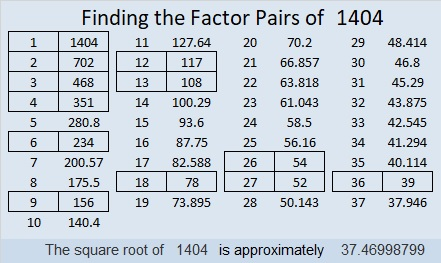1404 is the hypotenuse of a Pythagorean triple:
540-1296-1404 which is (5-12-13) times 108

Since 1404 has so many factors, it also has MANY different factor trees. Here are four of them mixed in with some Texas tessellations!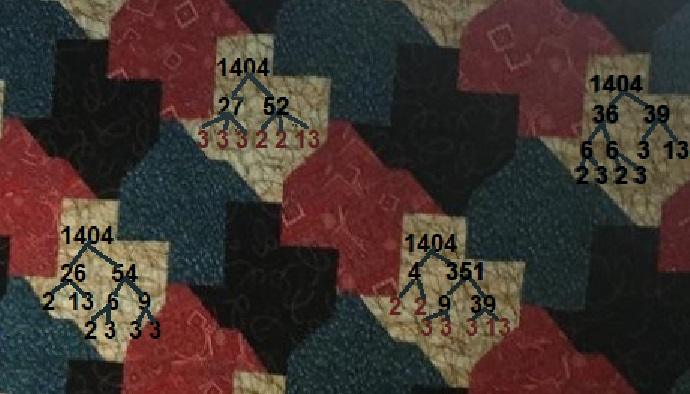# 656 Halloween Tangram Puzzle Cats

It’s almost Halloween, and I was in the mood to make some cats out of tangram pieces. MANY cat tangram patterns exist as well as patterns for witches hats, bats, haunted houses, and candles. Black makes the shapes look like shadows so I made a couple of patterns here (using excel).Tangram puzzles are made from the seven shapes in this square: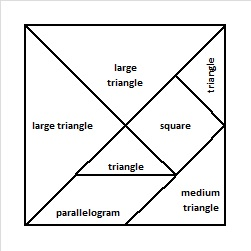How to fold and cut the tangram shapes from a half-sheet of black construction paper or other paper. (Thank you Paula Beardell Krieg from Bookzoompa.wordpress.com for the link on making a square and advice on the rest of the directions):

1. First make a square from the rectangular piece of paper, and visualize that square becoming the labeled square above.
2. Fold the square from the top left corner to the bottom right corner and crease the paper on the fold. Unfold the paper.
3. Partially fold the square from the top right corner to the bottom left corner allowing only the left half of the square to show a crease. This crease and the previous crease will form the two large triangles.
4. Fold the bottom right corner to the point where the other creases intersect. This new crease will form the medium triangle. Unfold. Cut out the medium triangle.5. Refold the top right corner to the bottom left corner and crease the entire length this time.
6. Cut along all the creases. You will then have cut out the two large triangles and be left with two odd pieces that look like this: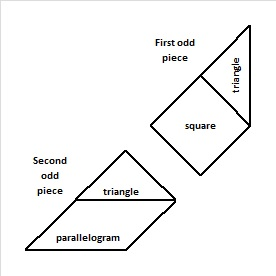7. Take the first odd piece and fold the longest straight edge so its endpoints meet. Crease and unfold. You should see a square and a small triangle. Cut them out.
8. Place the newly cut small triangle over the second odd piece so that you see a small triangle and a parallelogram. Cut along the bottom edge of the small triangle so that you have a second small triangle and a parallelogram.

Now all seven pieces have been cut out and you can make your own cat or other fun creation!

———————————————————-

Here are some facts about the number 656:

656 is a palindrome in three bases:

• 220022 in base 3; Note: 2(243) + 2(81) + 0(27) + 0(9) + 2(3) + 2(1) = 656
• 808 in base 9; Note: 8(81) + 0(9) + 8(1) = 656
• 656 in base 10; Obviously, 6(100) + 5(10) + 6(1) = 656

656 is the hypotenuse of the Pythagorean triple 144-640-656. What is the greatest common factor of those three numbers?

• 656 is a composite number.
• Prime factorization: 656 = 2 x 2 x 2 x 2 x 41, which can be written 656 = (2^4) x 41
• The exponents in the prime factorization are 4 and 1. Adding one to each and multiplying we get (4 + 1)(1 + 1) = 5 x 2 = 10. Therefore 656 has exactly 10 factors.
• Factors of 656: 1, 2, 4, 8, 16, 41, 82, 164, 328, 656
• Factor pairs: 656 = 1 x 656, 2 x 328, 4 x 164, 8 x 82, or 16 x 41
• Taking the factor pair with the largest square number factor, we get √656 = (√16)(√41) = 4√41 ≈ 25.612497.# 360 What Can You Do With Fraction Circles?

360 has more factors than any previous number. 240 and 336 held the previous record of 20 factors for each of them. How many factors do you think 360 has? Scroll down to the end of the post to find out.

360 can be evenly divided by every number from one to ten except seven, so it was a good number for the ancients to choose when they divided the circle into 360 degrees.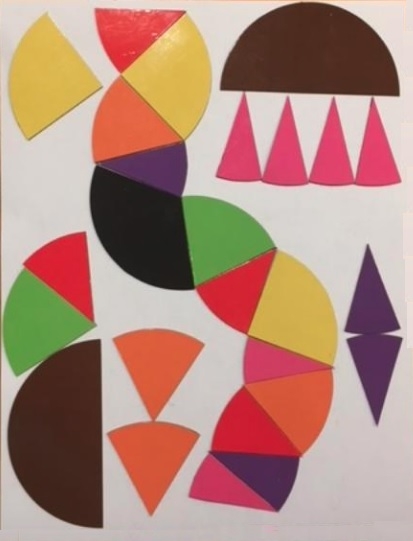I bought a few fraction circles. Each 51 piece set consists of 1 whole circle as well as circles divided into 2 halves, 3 thirds, 4 fourths, 5 fifths, 6 sixths, 8 eighths, 10 tenths, and 12 twelves. What can you do with fraction circles? You can do a lot with them no matter what your age.

Art and Mathematics

The fraction circle shapes can be used just as tangram shapes to create artwork, big or small. A couple of cool symmetric designs can be found at fraction-art and fraction-circle-art. Adding rectangular fraction pieces will increase the possibilities. Here are some simple artistic designs.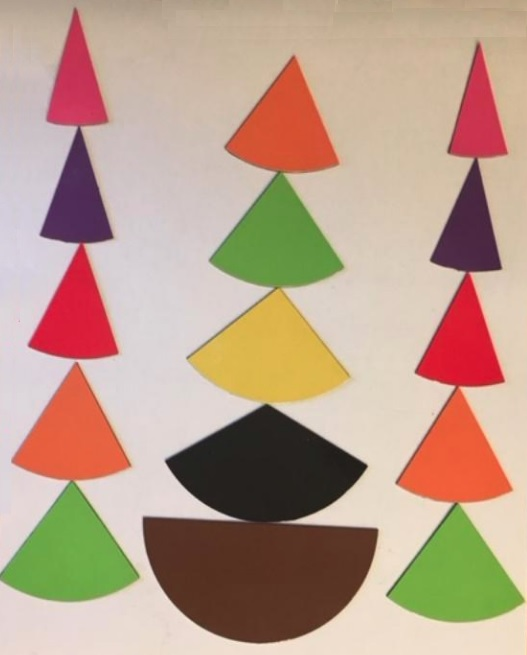Fraction Relationships

You can use fraction circle shapes to explore the relationship between fractions such as ½, ¼, and  ⅟₈;  ⅟₃, ⅟₆  and ⅟₁₂; or ½, ⅟₅ and ⅟₁₀: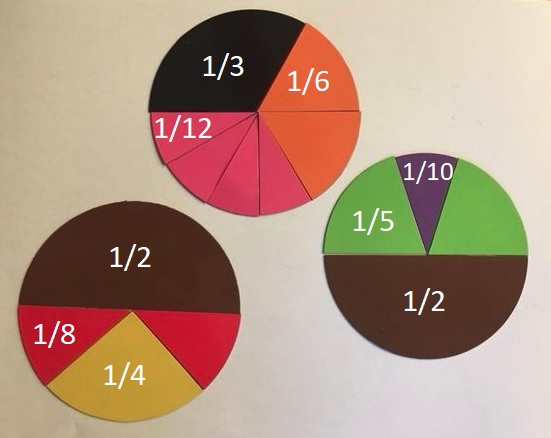Areas of Parallelograms, Trapezoids, and CirclesThe picture above shows what happens when the circle is divided into four, six, eight, ten or twelve equal wedges, and the wedges are arranged into something that resembles a parallelogram. This idea can be so easily duplicated with these fraction circles without any cutting.

Here are some good questions to ask:

1. What happens to the top and bottom of the shape when the number of wedges increases?
2. Sometimes the resulting shape will look like a trapezoid, and sometimes it looks more like a parallelogram. Why does that happen?We know that the circumference of any circle is 2πr with π defined as the circumference divided by the radius. π is the same value no matter how big or small the circle is.

We can calculate the area of any of the parallelogram-like shapes or trapezoid-like shapes above. Let’s call the length of the bottom of the shape b₁ and the length of the top b₂. The area of the resulting shape is calculated: A = ½ · (b₁ + b₂) · h. Since b₁ + b₂ = 2πr, and the height equals the radius, we can write our formula for the area of a circle as A = ½ · 2πr · r = πr².

This exercise demonstrates that the area of rectangles, parallelograms, trapezoids, and circles are all related!

Introduction to Pie Charts

Pie charts are a great way to display data when we want to look at percentages of a whole. If you use fraction circles, you are limited to using only to certain percentages, but they can still make a good introduction to the subject. To make the pie chart work either the total of all the degrees will have to equal 360 or the total of all the percents will have to equal 100:After a brief introduction using the fraction circles, try Kids Zone Create a Graph. It’s really easy to use!

Exploring Perimeter and Introducing Radians in Trigonometry

The perimeter of each fraction circle piece can be calculated. If the r = 1, the circumference of the circle is 2π, and we can see an important relationship between the degrees and the perimeter of each piece.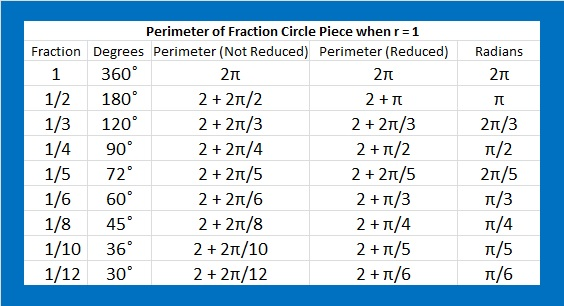What experiences have YOU had with circle fractions? Did you find them frustrating or enlightening? Personally, I like them very much, but I wish they had also been cut into ninths.

Here are some facts about the number 360:

The interior angles of every convex or concave quadrilateral total 360 degrees.

The exterior angles of every convex or concave polygon also total 360 degrees.

Here is all the factoring information about 360:

• 360 is a composite number.
• Prime factorization: 360 = 2 x 2 x 2 x 3 x 3 x 5, which can be written 360 = 2³·3²·5
• The exponents in the prime factorization are 3, 2 and 1. Adding one to each and multiplying we get (3 + 1)(2 + 1)(1 + 1) = 4 x 3 x 2 = 24. Therefore 360 has exactly 24 factors.
• Factors of 360: 1, 2, 3, 4, 5, 6, 8, 9, 10, 12, 15, 18, 20, 24, 30, 36, 40, 45, 60, 72, 90, 120, 180, 360
• Factor pairs: 360 = 1 x 360, 2 x 180, 3 x 120, 4 x 90, 5 x 72, 6 x 60, 8 x 45, 9 x 40, 10 x 36, 12 x 30, 15 x 24 or 18 x 20
• Taking the factor pair with the largest square number factor, we get √360 = (√10)(√36) = 6√10 ≈ 18.974